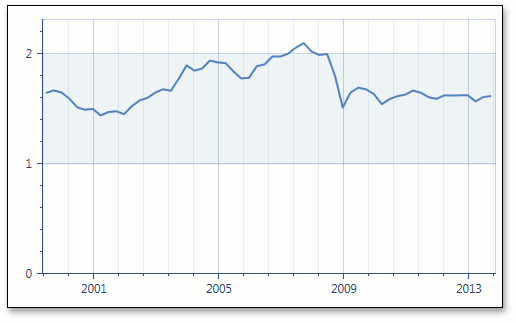# ManualDateTimeScaleOptions.AggregateFunction Property

Gets or sets the function that is used to aggregate date-time axis data when one of the AxisX2D.DateTimeScaleOptions, AxisX3D.DateTimeScaleOptions, or RadarAxisX2D.DateTimeScaleOptions properties is set to ManualDateTimeScaleOptions.

Namespace: DevExpress.Xpf.Charts

Assembly: DevExpress.Xpf.Charts.v23.1.dll

NuGet Package: DevExpress.Wpf.Charts

## Declaration

``public AggregateFunction AggregateFunction { get; set; }``

## Property Value

Type Description
AggregateFunction

An AggregateFunction enumeration value.

Available values:

Name Description
None

The aggregate function is not applied.

Average

Aggregates data by calculating the average value for a selected numeric or date-time interval.

Minimum

Aggregates data by calculating the minimum value for a selected numeric or date-time interval.

Maximum

Aggregates data by calculating the maximum value for a selected numeric or date-time interval.

Sum

Aggregates data by calculating the summary for a selected numeric or date-time interval.

Count

Aggregates data by calculating the number of non-null values for a selected numeric or date-time interval.

Histogram

Aggregates data by calculating the number of all points for a selected numeric or date-time interval.

Financial

Aggregates financial data for a selected interval into a single high-low-open-close data point, so that the high value equals the highest value of all data points in this interval; the low value equals the lowest value of all data points; the open value equals the value of the first data point and the close value equals the value of the last data point.

Custom

Aggregates data using the custom CustomAggregateFunction calculator.

## Remarks

The image below shows date-time data aggregation in the Manual scale mode using the Maximum function.Note

The AggregateFunction property is set to AggregateFunction.Average by default.

To disable date-time data aggregation, set the AggregateFunction property to AggregateFunction.None.

## Example

This example demonstrates how to use the manual date-time scale options of the X-axis.

Data aggregation is enabled automatically for the date-time scale on the X-axis in both automatic and manual scale options.

When the AxisX2D.DateTimeScaleOptions property is set to ManualDateTimeScaleOptions, you can define the ManualDateTimeScaleOptions.GridAlignment, ManualDateTimeScaleOptions.MeasureUnit and `ManualDateTimeScaleOptions.AggregateFunction` properties manually.

To use the automatic date-time scale options, set the AxisX2D.DateTimeScaleOptions property to AutomaticDateTimeScaleOptions, and select the appropriate AutomaticDateTimeScaleOptions.AggregateFunction.

To prevent the axis scale from being divided into intervals (and thus prevent chart data from being aggregated), set the `ManualDateTimeScaleOptions.AggregateFunction` property to None.

``````<Window
xmlns="http://schemas.microsoft.com/winfx/2006/xaml/presentation"
xmlns:x="http://schemas.microsoft.com/winfx/2006/xaml"
xmlns:dxc="http://schemas.devexpress.com/winfx/2008/xaml/charts"
x:Class="WpfApplication19.MainWindow"
Title="MainWindow" Height="400" Width="525">
<Grid>
<dxc:ChartControl>
<dxc:XYDiagram2D >
<dxc:XYDiagram2D.AxisX>
<dxc:AxisX2D GridLinesMinorVisible="True" GridLinesVisible="True">
<dxc:AxisX2D.DateTimeScaleOptions>
<dxc:ManualDateTimeScaleOptions AggregateFunction="Maximum" AutoGrid="False"
GridAlignment="Year" MeasureUnit="Month"
GridSpacing="3" GridOffset="1"/>
</dxc:AxisX2D.DateTimeScaleOptions>
</dxc:AxisX2D>
</dxc:XYDiagram2D.AxisX>
<dxc:LineSeries2D DataSource="{Binding Path=Rate}"
ArgumentDataMember="Argument" ValueDataMember="Value"  />
</dxc:XYDiagram2D>
</dxc:ChartControl>
</Grid>
</Window>
``````